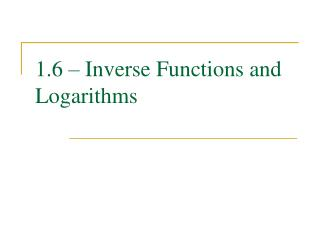DownloadDownload Presentation1.6 – Inverse Functions and Logarithms

# 1.6 – Inverse Functions and Logarithms

Download Presentation## 1.6 – Inverse Functions and Logarithms

- - - - - - - - - - - - - - - - - - - - - - - - - - - E N D - - - - - - - - - - - - - - - - - - - - - - - - - - -
##### Presentation Transcript

1. 1.6 – Inverse Functions and Logarithms

2. One-To-One Functions A function is one-to-one if no two domain values correspond to the same range value. Algebraically, a function is one-to-one if f (x1) ≠ f (x2) for all x1 ≠ x2. Graphically, a function is one-to-one if its graph passes the horizontal line test. That is, if any horizontal line drawn through the graph of a function crosses more than once, not it is one-to-one.

3. Try This Determine if the following functions are one-to-one. (a) f (x) = 1 + 3x – 2x 4 (b) g(x) = cos x + 3x 2 (c) (d)

4. Inverse Functions The inverse of a one-to-one function is obtained by exchanging the domain and range of the function. The inverse of a one-to-one function f is denoted with f -1. Domain of f = Range of f -1 Range of f = Domain of f-1 f −1(x) = y<=> f (y) = x

5. Try This Sketch a graph of f (x) = 2x and sketch a graph of its inverse. What is the domain and range of the inverse of f. Domain: (0, ∞) Range: (-∞, ∞)

6. Inverse Functions You can obtain the graph of the inverse of a one-to-one function by reflecting the graph of the original function through the line y = x.

7. Inverse of a One-To-One Function • To obtain the formula for the inverse of a function, do the following: • Let f (x) = y. • Exchange y and x. • Solve for y. • Let y = f −1(x).

8. Inverse Functions Determine the formula for the inverse of the following one-to-one functions. (a) (b) (c)

9. Logarithmic Functions The inverse of an exponential function is called a logarithmic function. Definition: x = a y if and only if y = log ax

10. Logarithmic Funcitons The function f (x) = log ax is called a logarithmic function. Domain: (0, ∞) Range: (-∞, ∞) Asymptote:x = 0 Increasing for a > 1 Decreasing for 0 < a < 1 Common Point: (1, 0)

11. Logarithmic Functions Now determine the inverse of g(x) = 3x. Definition: x = a y if and only if y = log ax

12. Properties of Logarithms • log a (ax) = x for all x  • alog a x = x for all x > 0 • log a (xy) = log ax + log a y • log a(x / y) = log ax – log a y • log axn = nlog ax • loga ax = x Common Logarithm: log 10x = log x Natural Logarithm: log ex = ln x All the above properties hold.

13. Properties of Logarithms The natural and common logarithms can be found on your calculator. Logarithms of other bases are not. You need the change of base formula. where b is any other appropriate base.

14. Try These • Determine the exact value of log 8 2. • Determine the exact value of ln e2.3. • Evaluate log 7.3 5 to four decimal places. • Write as a single logarithm: ln x + 2ln y – 3ln z. • Solve 2x +5 = 3 for x.

15. Try This In the theory of relativity, the mass of a particle with velocity v is where m0 is the mass of the particle and c is the speed of light in a vacuum. Find the inverse function of f and explain its meaning.